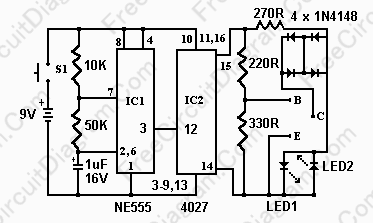# Simple Transistor Tester Using 555 ICTesting whether a transistor is shorted or open usually done by ohm meter. You test if the base to emitter  or collector permit a current flow in one direction. Here is the circuit’s schematic diagram:The 555 timer ( IC1 ) operate as a 12hz  oscillator. The output of this oscillator (pin 3) drives the 4027 flip-flop (IC2). Clocked by the oscillator, this flip-flop give complementary voltage outputs on pin 15 and 14. The outputs drives  LED1 and LED2 through the current limiting resistor R3. The LED’s are arranged so that if  the polarity across the circuit is one way then only one LED will light and if the polarity reverses then the other LED will light, and  when no transistor is connected to the tester then the LED’s will alternately flash. The IC2 outputs are also connected to resistors R4 and R5 with the junction of these two resistors connected to the base of the transistor being tested. If a good transistor connected to the tester, the transistor will turn on and produce a short across the LED pair. If a good NPN transistor is connected then  only LED1 will flash. If a good PNP transistor is connected then  only LED2 will flash. If the transistor is open both LED’s will flash and if the transistor is shorted then neither LED will flash.

After receiving a feedback from our reader, I’ve checked the datasheet of 4027 JK flip-flop, and found that the pin 12 and 13 in the schematic diagram above should be interchanged. Here is the feedback:

Hi

I am writing in reference to the circuit on the below link:
https://freecircuitdiagram.com/2008/08/21/simple-transistor-tester-using-555-ic/